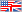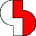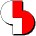Welcome to the Bartels Group of CompaniesBartels :: Bartels AutoEngineer :: BAE Documentation :: User Language Programmer's Guide :: Language Description :: Expressions
Bartels User Language - Programmer's Guide

# 2.4 ExpressionsThis section describes the expression operators provided by the Bartels User Language. The operators are introduced in a sequence according to decreasing operator precedence. The associativity, i.e., the order of evaluation is mentioned as well; each operator is evaluated either from the left to the right or vice versa (depending on the implicit parentheses). The precedence indicates the priority at which the corresponding expression is evaluated. The sequence of evaluation is undefined, unless the precedence takes effect. It is left up to the implementation, to evaluate partial expressions in the most efficient way, even if these expressions cause side effects. The evaluation sequence of the side effects thereby is undefined. Expressions with associative and/or commutative operators can be rearranged arbitrarily. Certain sequences of operator evaluation can be forced by assignments to (temporary) variables.

## 2.4.1 Primary Expressions

Primary expressions include constants, object references, parenthesis-enclosed expressions, function calls, array element accesses, structure element accesses or index element accesses. These operations are left-associative, i.e., they are evaluated from left to right.

#### Constants and Object References

Each suitable arranged identifier referring to an object is a valid primary expression. Integer constants, floating point constants, character constants and string constants are primary expressions as well (see chapter 2.2.3 for the representation of constants).

#### Parenthesis-Enclosed Expressions

An expression enclosed with parentheses is a primary expression. Parentheses can be set explicitly to redefine the sequence of evaluation. Since the multiplicative operators have higher precedence than the additive operators, the resulting value of the expression in

`a + b * c`

emerges from the sum of variable a plus the product of b*c, while the resulting value of the expression in

`(a + b) * c`

emerges from the product of the sum a+b multiplied by the variable c.

#### Function Call

A function call consists of the name of the function to be called, followed by a parenthsis-enclosed list of comma-separated expressions. The values of the expression list represent the current parameters for the function call. A function call can be used as primary in any other expression, if the corresponding function returns a non-void value. Examples for typical function calls are

```init_states(pass=1);
printf("This is a message!\n");
printf("Element %s\n",bae_planename());
```

#### Array Element Access

A primary expression followed by a bracket-enclosed expression again is a primary expression. This operation applies for the access to an array (or `string`) element. The expression left to the brackets refers to the array; the expression enclosed with the brackets is interpreted as `int` array index value indicating the required array element. The index value must not be negative, and the index value 0 (zero) refers to the first element of the array. When storing to an array, the User Language Interpreter automatically adjusts the array length limit and redefines the currently valid array range if necessary. Read access to an array is only permitted in the defined array range. In the following example the `strisoctal` function checks, whether the given `string` value contains octal digits only (`0` to `7`), and returns 1 if so or zero otherwise:

```int strisoctal(string str)
{
for (i=0;i<strlen(str);i++)
if (!isdigit(str[i]) || str[i]=='8' || str[i]=='9')
return(0);
return(1);
}
```

In the example above, the array range is checked with the strlen system function. The following example uses a special `int` variable ("filecount") for performing the array range check:

```string curfilename="", filelist[];
int i, filecount=0;
while (scandirfnames(".",".ddb",curfilename)==1)
filelist[filecount++]=curfilename;
for (i=0;i<filecount;i++)
printf("File %s \n",filelist[i]);
```

Within the example above first a list of file names (to be found in the current directory and ending on `.ddb`) is build, and then this file name list is printed.

#### Structure and Index Element Access

A primary expression followed by a point and an identifier again is a primary expression. The expression left to the point refers to a structure or `index` type, and the identifier following to the point operator designates a defined element of the corresponding structure or `index` type. The write access to structure elements is always possible, but no storage is permitted on `index` type elements (this would cause the User Language Compiler to issue a corresponding error message). The read access to the elements of a currently valid `index` variable always is permissible, while only previously initialized `struct` variable elements can be read (otherwise the User Language Interpreter would encounter a memory access violation and issue a corresponding error message). The following program part defines a list of structures and produces for each macro of the currently loaded layout a list element containing the macro name and the macro class:

```int macrocnt=0;
struct { string name; int class; } macrolist[];
index L_MACRO macro;
forall (macro) {
macrolist[macrocnt].name=macro.NAME;
macrolist[macrocnt++].class=macro.CLASS;
}
```

## 2.4.2 Unary Expressions

Unary expressions include all operators evaluating a single operand. These operations are right-associative, i.e., they are evaluated from right to left.

#### Increment and Decrement

The unary increment operator `++` changes its operand by adding the value 1 to the value of the operand. The unary decrement operator `--` changes its operand by subtracting the value 1 from the operand. The increment and decrement operators can be used either as prefix operator as in `++n` or as postfix operator as in `n++`. Both the prefix and the postfix notation cause an increment and/or decrement of the operand. The difference however is that the prefix expression changes the operand before using its value, while the postfix expression changes the operand after using its value. I.e., the result of these expression can have different meanings according to the program context. If, e.g., the value of `count` is 12, then

`n = --count ;`

sets the value of `n` to 11, but

`n = count-- ;`

sets the value of `n` to 12 (in both cases the value of `count` becomes 11).

#### Arithmetic Negation

The resulting value of the unary operator `-` is the arithmetic negation of its operand, i.e., the operand's value multiplied by (-1).

#### Logical Negation

The resulting value of the unary operator `!` is the logical negation of its operand. The value is set to either 1 for zero operand values (i.e., 0 or empty string for `string` operands), or 0 for nonzero operand values. The type of the result is `int`.

#### Bit Complement

The unary operator `~` yields the one's-complement of its operand. The operand must be of type `int`; each 1-bit of the operand is converted to a 0-bit and vice versa.

## 2.4.3 Binary Expressions

Binary expressions include all operators evaluating two operands. These operations are left-associative, i.e., they are evaluated from left to right.

#### Product

The operators for multiplication and division produce a product expression. The usual arithmetic type conversions are performed.

The binary `*` operator indicates multiplication. The resulting value of this operation is the product of its two operands. The multiplication operator is associative and commutative, and expressions with several multiplications at the same level can be rearranged arbitrarily.

The binary `/` operator indicates division. The resulting value emerges from dividing the first operand (dividend) by the second operand (divisor). Integer division truncates any fractional part. A zero value is not allowed for the divisor since division by zero is not permissible.

The binary `%` (modulus) operator yields the remainder of dividing the first operand (dividend) by the second operand (divisor); floating point operand values are not allowed, and the divisor must not be zero. The `%` operator can be utilized as in

```febdays = (year%4==0 && year%100!=0 || year%400==0) ? 29 : 28 ;
```

where the value of `year` is tested on leap-year match, and the value of `febdays` is set accordingly.

#### Sum

The operators for addition and subtraction produce a sum expression. The usual type conversions are performed.

The binary `+` (plus) operator indicates addition. If this operator is applied on numerical operands, then the result is the sum of its two operands, and the operation is commutative; if the add operator is applied on `string` operands, then the result is a string value generated by appending the second `string` to the first `string`, and the operation is not commutative.

The binary `-` (minus) operator indicates subtraction. The resulting value of this operation is the difference of its operands. The second operand is subtracted from the first.

#### Shift Operation

The binary shift operators `<<` and `>>` can be applied to integer operands. They perform a left (`<<`) or right (`>>`) shift of their first operand by the number of bit positions specified with the second operand. Vacated significant bits of the first operand are filled with 0-bits. The result value of a bit-shift operation is undefined, if the second operand is negative. If the second operand is zero (i.e., 0 shift operations requested), then the first operand leaves unchanged. A right-shift by one bit corresponds with a (fast) integer division by 2; a left-shift by one bit corresponds to a (fast) multiplication by two; a left-shift by two bits corresponds with a multiplication by four, etc.

#### Comparison

The resulting value of the binary comparison operators `<` (less than), `<=` (less equal), `>` (greater than) and `>=` (greater equal) is the `int` value 1, if the specified comparison relation is true for the two operands; otherwise the result value is 0. The comparison operators can be applied on `string` operands directly.

#### Equivalence

The resulting value of the binary equivalence operators `==` (equal) and `!=` (not equal) is the `int` value 1, if the specified equality relation is true for the two operands; otherwise the result value is 0. The equivalence operators can be applied on `string` operands directly; they correspond to the comparison operators, but they have less precedence.

#### Bitwise AND

The binary bitwise AND operator `&` applies to integer operands only; the usual arithmetic type conversions are performed. The result is the bitwise AND function of its operands. This operator is associative and commutative and expressions involving `&` can be rearranged.

#### Bitwise Exclusive OR

The binary bitwise exclusive OR operator `^` applies to integer operands only; the usual arithmetic type conversions are performed. The result is the bitwise exclusive OR (XOR) function of its operands. This operator is associative and commutative, and expressions involving `^` can be rearranged.

#### Bitwise Inclusive OR

The binary bitwise inclusive OR operator `|` applies to integer operands only; the usual arithmetic type conversions are performed. The result is the bitwise inclusive OR function of its operands. This operator is associative and commutative, and expressions involving `|` can be rearranged.

#### Logical AND

The logical AND operator `&&` returns the `int` value 1 if both its operands are nonzero or 0 otherwise. This operator strictly guarantees left-to-right evaluation; i.e., the second operand is not evaluated if the value of the first operand is zero, such that in an expression like

`x<100 && fct(x)`

the `fct` function is only called if the value of `x` is less than 100.

#### Logical OR

The logical OR operator `||` returns the `int` value 1 if either of its its operands is nonzero or 0 otherwise. This operator strictly guarantees left-to-right evaluation; i.e. the second operand is not evaluated if the value of the first operand is nonzero, such that in an expression like

`test1() || test2()`

the `test2` function is only called if the `test1` function returns zero.

#### Conditional Evaluation

The ternary operator `?:` is the conditional evaluation; this operation is right-associative, i.e., it is evaluated from right to left. The first expression is evaluated and if it is nonzero, the result is the value of the second expression, otherwise that of third expression. Usual type conversions are performed to bring the second and third expressions to a common type. Only one of the second and third expression is evaluated. An expression for assigning a conditional expression value to a result as in

`result = logexpr ? trueexpr : falsexpr ;`

is equivalent to the following control structure:

```if (logexpr)
result = trueexpr ;
else
result = falseexpr ;
```

The following example utilizes the conditional expression operator to calculate the maximum of two values:

`maxval = (val1>=val2) ? val1 : val2 ;`

#### Assignments

User Language provides a series of assignment operators, all of which are right-associative. All assignment operators require an unary expression as their left operand; the right operand can be an assignment expression again. The type of the assignment expression corresponds to its left operand. The value of an assignment operation is the value stored in the left operand after the assignment has taken place. The binary `=` operator indicates the simple assignment; the binary operators `*=`, `/=`, `%=`, `+=`, `-=`, `>>=`, `<<=`, `&=`, `^=` and `|=` indicate a compound assignment expression. A compound assignment of general form as in

`expr1 <operator>= expr2 `

is equivalent with the expression

`expr1 = expr1 <operator> (expr2)`

where, however, expr1 is evaluated only once (consider the parentheses round expression expr2). An assignment expression sequence as in

```a = 5 ;
b = 3-a ;
c = b+a ;
c -= a *= b += 4+2*a ;
```

stores the values 60, 12 and -57 to the variables `a`, `b` and `c`, respectively.

## 2.4.4 Expression List

Each expression can consist of a list of comma-separated binary expressions. A pair of expressions separated by comma is evaluated left-to-right and the value of the left expression is discarded. The type and value of the result are the type and value of the right operand. The comma operator can be utilized as in

`c -= (a=5, b=3-a, c=b+a, a*=b+=4+2*a) ;`

where the values 60, 12 and -57 are stored to the variables `a`, `b` and `c`, respectively. In contexts where the comma is given a special meaning, e.g., in a list of actual function parameters and lists of initializers, the comma operator can only appear in parentheses; e.g., the function call

`fct ( x, (y=8, y*25.4), z )`

has three arguments, the second of which has the value 203.2.

## 2.4.5 Precedence and Order of Evaluation

Table 2-4 summarizes the rules for precedence and associativity of all User Language operators. Operators on the same line have the same precedence; rows are in order of decreasing precedence.

Table 2-4: Operator Precedence and Order of Evaluation

OperationOperator(s)Associativity
Primary () [] . left to right
Unary ! ~ ++ -- - right to left
Product * / % left to right
Sum + - left to right
Shift << >> left to right
Comparison < <= > >= left to right
Equality == != left to right
Bit And & left to right
Bit Xor ^ left to right
Bit Or | left to right
Logical And && left to right
Logical Or || left to right
Conditional ?: right to left
Assignment = += -= etc. right to left
Expression List, left to right
 Bartels :: Bartels AutoEngineer :: BAE Documentation :: User Language Programmer's Guide :: Language Description :: Expressions

Expressions
© 1985-2022 Oliver Bartels F+E • Updated: 05 December 2006, 16:54 [UTC]© 1985-2022 Oliver Bartels F+E Bartels Homepage Contact and Corporate InfoWeb Development by Baumeister Mediasoft Engineering